Next: Source as a Sum Up: RADIATION: MATHEMATICAL RELATION TO Previous: Unit Impulse Response

## Full Scalar Radiation Field

The scalar field measured in Rindler sectorof the expanding inertial reference frame is the linear superposition
(44)

of two contributions. They arise from two causally disjoint sources, one in Rindler sector
, the other in Rindler sector. Applying Eq.(41) together with Eqs.(39) and (40) to Eq.(35), one finds that these contributions are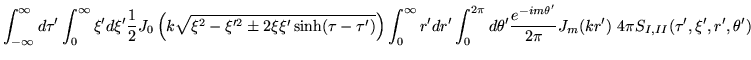(45)

These radiation fields are exact in the sense that we have made no assumptions about the size of the sources in relation to their radiated wavelengths. The only restriction is that the two source functions
andbe non-zero only inandrespectively.

The key to identifying the nature of these fields is the evaluation of the mode sum over
and the mode integral over
. These evaluations have been done already starting with Eq.(42), and gave rise to the familiar causal unit impulse response, Eq.(43). However, for the purpose of physical transparency we shall not make these evaluations. Instead, we shall make an evaluation which is based on the assumption that each source is small compared to its radiated wave lengths. Physically this means that the phase of the e.m. field is the same across the whole extent of the source. Mathematically this means that the Bessel function
should be replaced by the expression Eq.(46) below. The advantage of the long wave length approximation is physical/technological: it permits the characterization of the source in terms of multipole moments, which are directly tied to the angular distribution of the emitted radiation, and which derive their importance from, among others, the quantum mechanical selection rules.

SubsectionsNext: Source as a Sum Up: RADIATION: MATHEMATICAL RELATION TO Previous: Unit Impulse Response
Ulrich Gerlach 2001-10-09